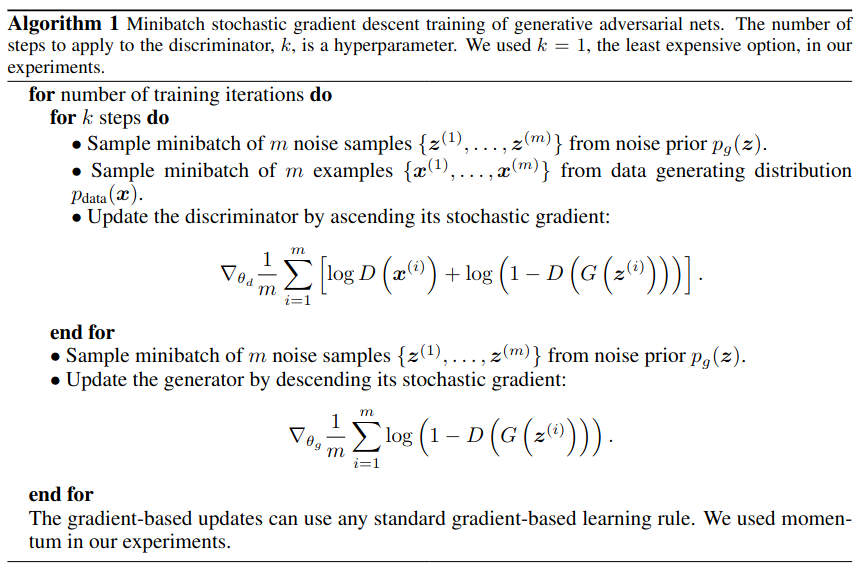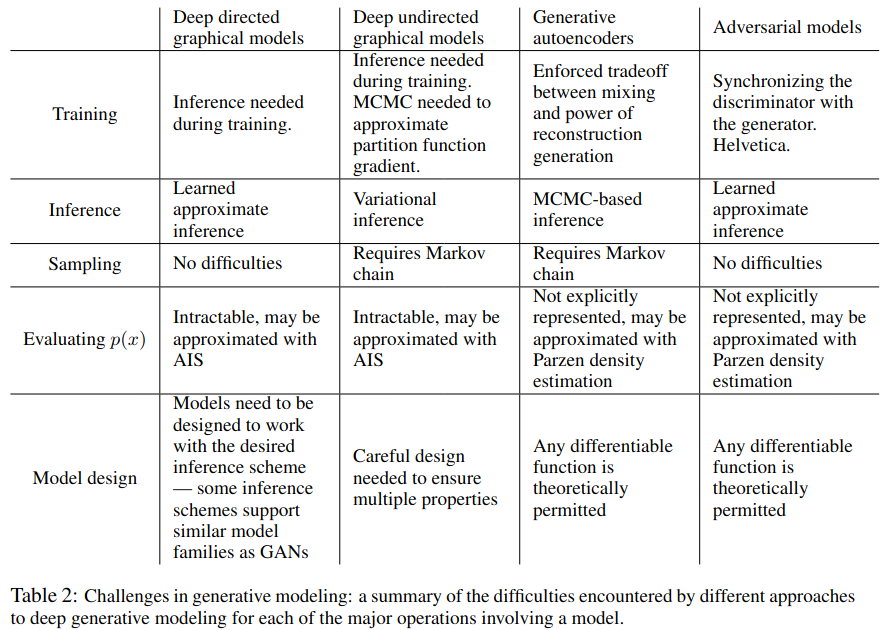## 2. 动机

$\lim _{\sigma \rightarrow 0} \nabla_{\boldsymbol{x}} \mathbb{E}_{\epsilon \sim \mathcal{N}\left(0, \sigma^{2} \boldsymbol{I}\right)} f(\boldsymbol{x}+\epsilon)=\nabla_{\boldsymbol{x}} f(\boldsymbol{x}) \tag{1}$

## 3. 方法

$\min _{G} \max _{D} V(D, G)=\mathbb{E}_{\boldsymbol{x} \sim p_{\text {data }}(\boldsymbol{x})}[\log D(\boldsymbol{x})]+\mathbb{E}_{\boldsymbol{z} \sim p_{\boldsymbol{z}}(\boldsymbol{z})}[\log (1-D(G(\boldsymbol{z})))] \tag{2}$

• 训练辨别器 $D$ 时，最大化式 $(2)$，此时会使用真实数据和 $p_g$ 生成的数据进行训练。当 $D$ 达到完美时，式 $(2)$ 中的两项都是 $\log{1} = 0$，否则就是一个负数，因此需要最大化式 $(2)$ 来优化 $D$

• 训练生成器 $G$ 时，最小化式 $(2)$，此时式 $(2)$ 的第一项相对于 $G$ 是常数，因此只需要最化化第二项。当 $G$ 达到完美时，$(2)$ 中的第二项为 $\log{0}$，即 $-\infty$，因此需要最小化式 $(1)$ 来优化 $G$

不过作者在实际中发现，最大化式 $(2)$ 中的第二项并不能很好地优化 $G$。于是作者建议在优化 $G$ 的时候可以把最小化式 $(2)$ 换成最大化 $\mathbb{E}_{\boldsymbol{z} \sim p_{\boldsymbol{z}}(\boldsymbol{z})}[\log D(G(\boldsymbol{z}))]$## 4. 证明

• 证明 GAN 在上述给定的算法下能求得最优解；
• 证明上述给定的算法能够收敛。

### 4.1 生成器的收敛性

$D^*_G(\boldsymbol{x}) = \frac{p_{data}(\boldsymbol{x})}{p_{data}(\boldsymbol{x}) + p_g(\boldsymbol{x})} \tag{3}$

\begin{aligned} V(G, D) &=\int_{\boldsymbol{x}} p_{\text {data }}(\boldsymbol{x}) \log (D(\boldsymbol{x})) d x+\int_{z} p_{\boldsymbol{z}}(\boldsymbol{z}) \log (1-D(g(\boldsymbol{z}))) d z \\ &=\int_{\boldsymbol{x}} p_{\text {data }}(\boldsymbol{x}) \log (D(\boldsymbol{x}))+p_{g}(\boldsymbol{x}) \log (1-D(\boldsymbol{x})) d x \end{aligned}

\begin{aligned} C(G) &=\max _{D} V(G, D) \\ &=\mathbb{E}_{\boldsymbol{x} \sim p_{\text {data }}}\left[\log D_{G}^{*}(\boldsymbol{x})\right]+\mathbb{E}_{\boldsymbol{z} \sim p_{\boldsymbol{z}}}\left[\log \left(1-D_{G}^{*}(G(\boldsymbol{z}))\right)\right] \\ &=\mathbb{E}_{\boldsymbol{x} \sim p_{\text {data }}}\left[\log D_{G}^{*}(\boldsymbol{x})\right]+\mathbb{E}_{\boldsymbol{x} \sim p_{g}}\left[\log \left(1-D_{G}^{*}(\boldsymbol{x})\right)\right] \\ &=\mathbb{E}_{\boldsymbol{x} \sim p_{\text {data }}}\left[\log \frac{p_{\text {data }}(\boldsymbol{x})}{P_{\text {data }}(\boldsymbol{x})+p_{g}(\boldsymbol{x})}\right]+\mathbb{E}_{\boldsymbol{x} \sim p_{g}}\left[\log \frac{p_{g}(\boldsymbol{x})}{p_{\text {data }}(\boldsymbol{x})+p_{g}(\boldsymbol{x})}\right] \end{aligned}

$C(G)=-\log (4)+K L\left(p_{\text {data }} \left\Vert \frac{p_{\text {data }}+p_{g}}{2}\right. \right)+K L\left(p_{g} \left\Vert \frac{p_{\text {data }}+p_{g}}{2}\right. \right) \tag{4}$

$C(G)$ 取到最小时即式 $(4)$ 中的两项 KL 散度均为 $0$，此时 $p_{data} = p_g$。故证明了在上述给定的算法框架下，生成器 $G$ 能收敛到最优值。

### 4.2 算法的收敛性

$V(G, D) = \mathbb{E}_{\boldsymbol{x} \sim p_{\text {data }}}\left[\log D_{G}^{*}(\boldsymbol{x})\right]+\mathbb{E}_{\boldsymbol{x} \sim p_{g}}\left[\log \left(1-D_{G}^{*}(\boldsymbol{x})\right)\right] \tag{5}$

## 5. 实验## 6. 总结## 附录

1. Goodfellow, I., Pouget-Abadie, J., Mirza, M., Xu, B., Warde-Farley, D., Ozair, S., ... & Bengio, Y. (2014). Generative adversarial nets. Advances in neural information processing systems, 27.